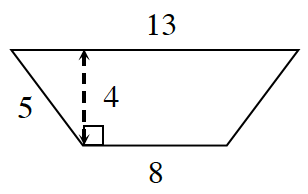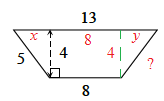### Home > CCG > Chapter 8 > Lesson 8.3.2 > Problem8-106

8-106.Use what you know about similar figures to complete the following tasks.

1. Find the area and perimeter of the trapezoid below.

Do not assume the trapezoid is isosceles even though it looks like it.
Start by solving for $x$ in the diagram, then solve for $y$, then calculate the length of the unknown side of the trapezoid.Area = $42$ square units

2. Find the area and perimeter of the trapezoid that is similar to this one, but has been reduced by a linear scale factor of $\frac { 1 } { 3 }$.